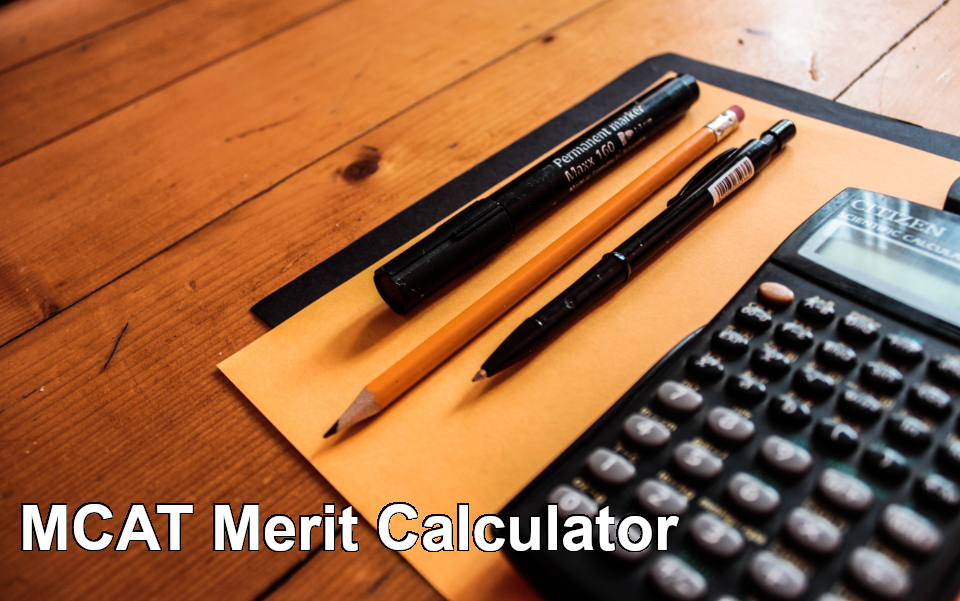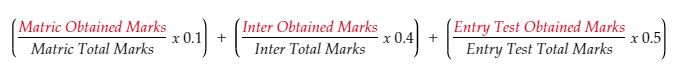# MDCAT (MCAT) Aggregate Calculator 2022 (Updated)Pakistan Medical Council (PMC) and UHS (University of Health Sciences) introduced a new/revised merit policy under COVID-19 for the year. And now the aggregate will be calculated with the new merit formula. MCAT merit calculator will calculate your aggregate based on your intermediate (or equivalent), matric (or equivalent), and entry test marks.
• Old Calculator
• New Calculator
Inter Obtained Marks
Inter Total Marks
Matric Obtained Marks
Matric Total Marks
Entry Test Obtained Marks
Entry Test Total Marks
You aggregate/merit:
(Inter 40%, Matric 10%, Entry Test 50%)
0.0
0
Metric Numbers
0
Inter Numbers
0
Entry Test Numbers
Inter Obtained Marks
Inter Total Marks
Entry Test Obtained Marks
Entry Test Total Marks
You aggregate/merit:
(Inter 50%, Entry Test 50%)
0.0
0
Inter Numbers
0
Entry Test Numbers

## Merit Formula

According to the new merit criteria merit is based on intermediate elective subjects numbers (Biology, Chemistry, Physics/Math) and entry test numbers:
• Intermediate (or equivalent) 50%
• Entry Test 50%
The general old formula for merit calculation was as follow:
• Matric (or equivalent) 10%
• Intermediate (or equivalent) 40%
• Entry Test 50%

## How to Calculate Aggregate?

To calculate the aggregate yourself just use this formula:## Updated Eligibility Criteria

For admission candidate will be evaluated on the following basis:
• Candidate should have 70% marks in intermediate (or equivalent)
• He/She must have passed MCAT with 60% marks
If you are not sure about your intermediate percentage check the following calculator and calculate your percentage.
Obtained Marks
Total Marks